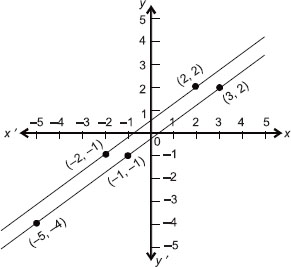# User ForumSubject :IMO    Class : Class 9

The equations representing the given graph is ______.A 7x + 2y = 11; y – 2x = 3
B 2x + 7y = 11; 5x + (35y/2) = 25
C 3x – 7y = 10; 8y – 6x = 4
D 3x – 4y = 1; 8y – 6x = 4

Class : Class 10

Class : Class 9